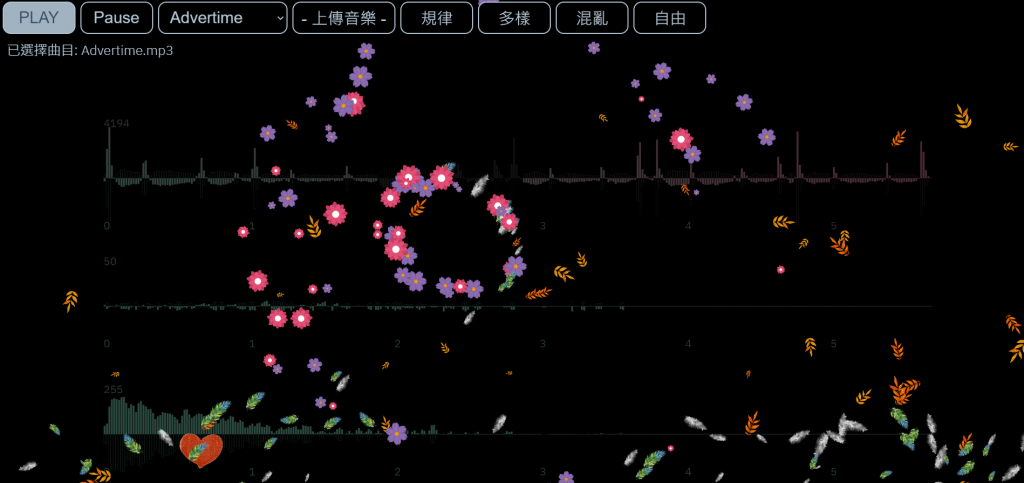#DAY 23
0
Modern Web

## Chapter4 - Canvas背景動畫（IV）把紛飛的落葉，通通抓回來當作收藏吧！https://jerry-the-potato.github.io/Chapter4-demo4/

A. 很想抓住它們
B. 很想收集起來
C. 很想控制風的流向
D. 其他想法？歡迎留言

## 碰撞檢測

1. 計算兩個物件中心點距離的平方: dx^2 + dy^2
2. 計算兩個物件半徑相加的平方: (r1 + r2)^2

``````animeObject.prototype.NextFrame = function(){

// 檢查是否與滑鼠碰撞
let distanceP2 = Math.pow(this.pointX - myMouse.pointX, 2) + Math.pow(this.pointY - myMouse.pointY, 2);
let mouseWidth = WIDTH * 0.05;
let thisWidth = this.sizeNow;
if(distanceP2 < Math.pow((mouseWidth * 0.4 + thisWidth * 0.5), 2)){
// 觸發收集的動畫
// ......
}

// 以下略
// ......
}
``````

`let myMouse = new mouseTrail(0, 0, true);`滑鼠幾乎每篇都有用到，這邊還是提醒一下，它是將第二章的學的滑鼠動畫，以第三章的觀念改寫成物件建構式，因為覺得沒有很重要，就沒拿來水文章了，若覺得困惑可以留言詢問。

``````let width = this.sizeNow;
let height = this.sizeNow * this.img.height / this.img.width;
context.drawImage(this.img, -width/2, -height/2, width, height);
``````

## 收集的動畫

1. 改變這個物件本身的狀態
2. 提供這個物件一股推力

``````animeObject.prototype.NextFrame = function(){
// 以上略
if(this.animeName == "Floating") this.Floating(dT);
else if(this.animeName == "Falling") this.Falling(dT);
else if(this.animeName == "Staring") this.Staring(dT);
// 以下略
}
``````

## 折衷的方案

「摁？你在工沙ㄒ...」別急著噴我！如果，今天是兩個看起來一模一樣的葉子出現在同一個位置，使用者分得出來嗎？叮咚！接下來我們要做的就是，複製一個看起來相同的葉子，來取代原本的葉子，如此一來，剛剛掉落的那片葉子就可以直接刪掉回收，也不用去動到原本我們設計的動畫物件了！

### 第二章學到的路徑動畫

``````// 路徑動畫物件
function animeObject2(img, size, originX, originY, targetX, targetY, frames = 120){
this.img = img;
this.sizeNow = size;
this.pointX = originX;
this.pointY = originY;
this.originX = originX;
this.originY = originY;
this.targetX = targetX;
this.targetY = targetY;
this.period = frames;
this.timer = frames;
}
``````

1. 製作一個新的葉子仿冒品
2. 刪掉原本的葉子
3. 將新的葉子放入原本的動畫清單中
``````if(distance2p < Math.pow((mouseWidth * 0.4 + thisWidth * 0.5), 2)){
// 用另一個物件取代該物件
let newObject = new animeObject2(this.img, this.sizeNow,
this.pointX, this.pointY,
WIDTH / 2, HEIGHT / 2, 120);
let index = animeList.indexOf(this);
delete animeList[index];
animeList[index] = newObject;
}
``````

``````function Redraw(){
clear(context);
AudioProcess();
MouseAnime();
animeList.forEach(obj => obj.NextFrame());
}
``````

``````animeObject2.prototype.NextFrame = function(){
if(this.timer > 0){
// 1. 計算座標
// 2. 渲染圖形
}
else{
// 3. 把動畫物件刪掉
}
}
``````

``````if(this.timer > 0){
// 1. 計算座標
let dX = this.targetX - this.originX;
let dY = this.targetY - this.originY;
let t = this.timer;
let p = this.period;
let linear = 1/p;
let easeout = Math.pow(t/p, 2) - Math.pow((t-1)/p, 2);
let easein = Math.pow(1 - (t-1)/p, 2) - Math.pow(1 - t/p, 2);
let a = 1;
let b = 1;
let c = -3;
this.pointX+= (a * linear + b * easein + c * easeout) / (a+b+c) * dX;
this.pointY+= (a * linear + b * easein + c * easeout) / (a+b+c) * dY;
this.timer--;
}
``````

### 半小時挑戰失敗XD，別急，讓我把code慢慢貼上來...

Yeah整理完了，結果花了兩小時撰寫文章、20分鐘校稿www